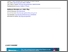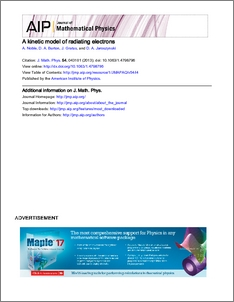# A kinetic model of radiating electrons

Noble, Adam and Gratus, Jonathan and Burton, David and Jaroszynski, Dino (2013) A kinetic model of radiating electrons. Journal of Mathematical Physics, 54 (4). ISSN 0022-2488Preview
PDF
ojpstmpstampPdf_d03T0918_JMAPAQ_54_4_043101_1.pdf - Published Version

## Abstract

A kinetic theory is developed to describe radiating electrons whose motion is governed by the Lorentz-Dirac equation. This gives rise to a generalized Vlasov equation coupled to an equation for the evolution of the physical submanifold of phase space. The pathological solutions of the $1$-particle theory may be removed by expanding the latter equation in powers of $\tau := q^2/ 6\pi m$. The radiation-induced change in entropy is explored, and its physical origin is discussed. As a simple demonstration of the theory, the radiative damping rate of longitudinal plasma waves is calculated.

Item Type:
Journal Article
Journal or Publication Title:
Journal of Mathematical Physics
Open Access Content (see the article's web page).
Uncontrolled Keywords:
/dk/atira/pure/researchoutput/libraryofcongress/qc
Subjects:
Departments:
ID Code:
59389
Deposited By:
Deposited On:
22 Oct 2012 15:59
Refereed?:
Yes
Published?:
Published